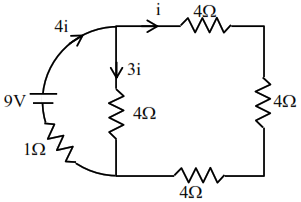# Solve thisQuestion:

A $16 \Omega$ wire is bend to form a square loop. A $9 \mathrm{~V}$ supply having internal resistance of $1 \Omega$ is connected across one of its sides. The potential drop across the diagonals of the square loop is ____________$\times 10^{-1} \mathrm{~V}$

Solution:

here assume current asBy KVL in outer loop

$9-12 i-4 i=0$

$16 i=9$

$8 i=\frac{9}{2}=4.5$

$=45 \times 10^{-1}$